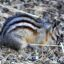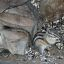﻿ Results IIISSAC 94 - Symbolic-Numeric Nonlinear Equation Solving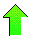Contents News View

 Results II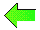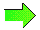The two dimensional algorithm has been implemented in Maple and run on some inputs including(1)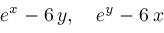for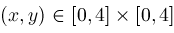(2)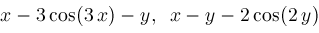foritemize Figures 4.1-4.2 plot the curves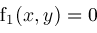and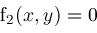for these examples.  The solutions being sought are the intersection points of these curves.

The operations of the two dimensional Zeros and Narrow procedures are represented graphically in Figures 5.1-5.2.  Most rectangles represent regions of the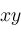-plane eliminated by steps in either Zeros or Narrow.  Only the very smallest rectangles represent regions that have not been eliminated and these surround the solutions we seek.

Finally, Figures 6.1-6.2 are closeups for selected solutions of these two systems which picture the trajectories of approximate solutions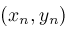computed by two dimensional versions of the Locate and Newton procedures.  The Newton iteration formulas for example (1) are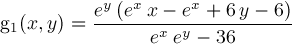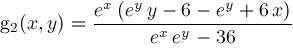and these lead to solution (x,y)=(2.8331478920493421425, 2.8331478920493421425) as shown in Figure 6.1